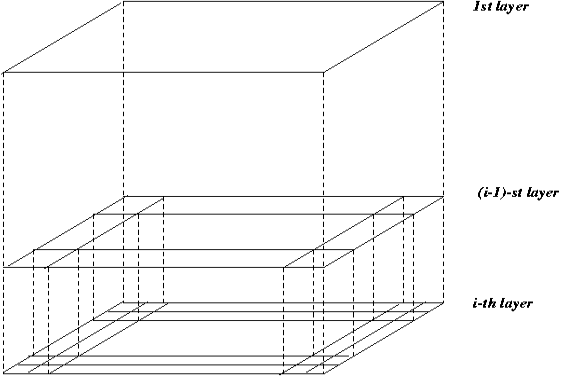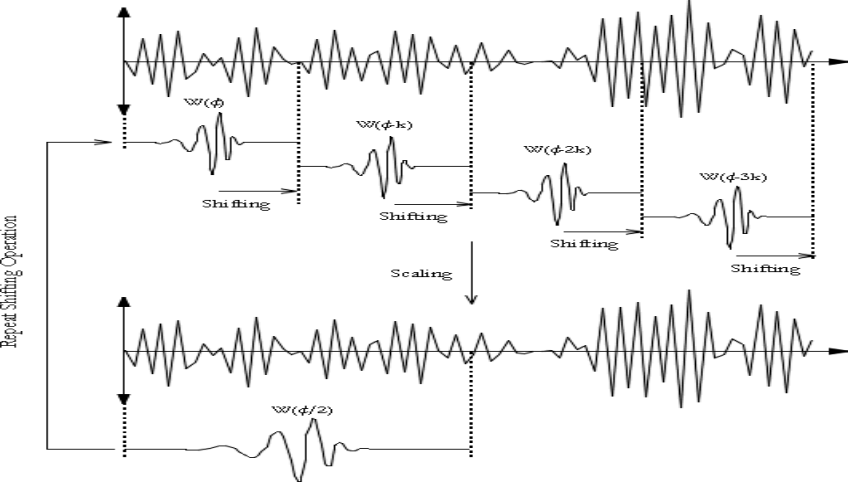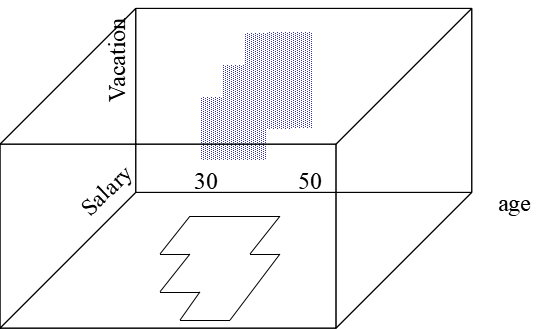# Grid-Based Clustering - STING, WaveCluster & CLIQUE

## Grid-Based Clustering

Grid-Based Clustering method uses a multi-resolution grid data structure.

(Partitional Clustering Methods - read here)

(Hierarchical Clustering Methods - read here)

(Density-Based Clustering Methods - read here)

Several interesting methods
• STING (a STatistical INformation Grid approach) by Wang, Yang, and Muntz (1997)
• WaveCluster by Sheikholeslami, Chatterjee, and Zhang (VLDB’98) - A multi-resolution clustering approach using wavelet method
• CLIQUE - Agrawal, et al. (SIGMOD’98)

## STING - A Statistical Information Grid Approach

STING was proposed by Wang, Yang, and Muntz (VLDB’97).

In this method, the spatial area is divided into rectangular cells.

There are several levels of cells corresponding to different levels of resolution.For each cell, the high level is partitioned into several smaller cells in the next lower level.

The statistical info of each cell is calculated and stored beforehand and is used to answer queries.

The parameters of higher-level cells can be easily calculated from parameters of lower-level cell
• Count, mean, s, min, max
• Type of distribution—normal, uniform, etc.
Then using a top-down approach we need to answer spatial data queries.

Then start from a pre-selected layer—typically with a small number of cells.

For each cell in the current level compute the confidence interval.

Now remove the irrelevant cells from further consideration.

When finishing examining the current layer, proceed to the next lower level.

Repeat this process until the bottom layer is reached.

It is Query-independent, easy to parallelize, incremental update.

O(K), where K is the number of grid cells at the lowest level.

All the cluster boundaries are either horizontal or vertical, and no diagonal boundary is detected.

## WaveCluster

It was proposed by Sheikholeslami, Chatterjee, and Zhang (VLDB’98).

It is a multi-resolution clustering approach which applies wavelet transform to the feature space
• A wavelet transform is a signal processing technique that decomposes a signal into different frequency sub-band.
It can be both grid-based and density-based method.

Input parameters:
• No of grid cells for each dimension
• The wavelet, and the no of applications of wavelet transform.How to apply the wavelet transform to find clusters
• It summaries the data by imposing a multidimensional grid structure onto data space.
• These multidimensional spatial data objects are represented in an n-dimensional feature space.
• Now apply wavelet transform on feature space to find the dense regions in the feature space.
• Then apply wavelet transform multiple times which results in clusters at different scales from fine to coarse.

Why is wavelet transformation useful for clustering
• It uses hat-shape filters to emphasize region where points cluster, but simultaneously to suppress weaker information in their boundary.
• It is an effective removal method for outliers.
• It is of Multi-resolution method.
• It is cost-efficiency.

Major features:
• The time complexity of this method is O(N).
• It detects arbitrary shaped clusters at different scales.
• It is not sensitive to noise, not sensitive to input order.
• It only applicable to low dimensional data.

## CLIQUE - Clustering In QUEst

It was proposed by Agrawal, Gehrke, Gunopulos, Raghavan (SIGMOD’98).

It is based on automatically identifying the subspaces of high dimensional data space that allow better clustering than original space.

CLIQUE can be considered as both density-based and grid-based:
• It partitions each dimension into the same number of equal-length intervals.
• It partitions an m-dimensional data space into non-overlapping rectangular units.
• A unit is dense if the fraction of the total data points contained in the unit exceeds the input model parameter.
• A cluster is a maximal set of connected dense units within a subspace.

Partition the data space and find the number of points that lie inside each cell of the partition.

Identify the subspaces that contain clusters using the Apriori principle.

Identify clusters:
• Determine dense units in all subspaces of interests.
• Determine connected dense units in all subspaces of interests.

Generate minimal description for the clusters:
• Determine maximal regions that cover a cluster of connected dense units for each cluster.
• Determination of minimal cover for each cluster.It automatically finds subspaces of the highest dimensionality such that high-density clusters exist in those subspaces.

It is insensitive to the order of records in input and does not presume some canonical data distribution.

It scales linearly with the size of input and has good scalability as the number of dimensions in the data increases.